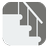Stair Steps
Elementary+
English RU

There is a staircase with N steps and two platforms; one at the beginning, the other at the end of the stairs. On each step a number is written (ranging from -100 to 100 with the exception of 0.) Zeros are written on both platforms. You start going up the stairs from the first platform, to reach the top on the second one. You can move either to the next step or to the next step plus one. You must find the best path to maximize the sum of numbers on the stairs on your way up and return the final sum.Input: Numbers on each stair as a list of integers.

Output: The final sum for the best way as an integer.

Example:

```checkio([5, -3, -1, 2]) == 6
checkio([5, 6, -10, -7, 4]) == 8
checkio([-11, 69, 77, -51, 23, 67, 35, 27, -25, 95]) == 393
checkio([-21, -23, -69, -67, 1, 41, 97, 49, 27]) == 125```

How it is used: This is a classical example of the optimisation problem. It can show you the difference between the various methods of programming; such as dynamic programming and recursion.

Precondition:
0 < len(steps) ≤ 10
all(-100 < x < 100 and x for x in steps)